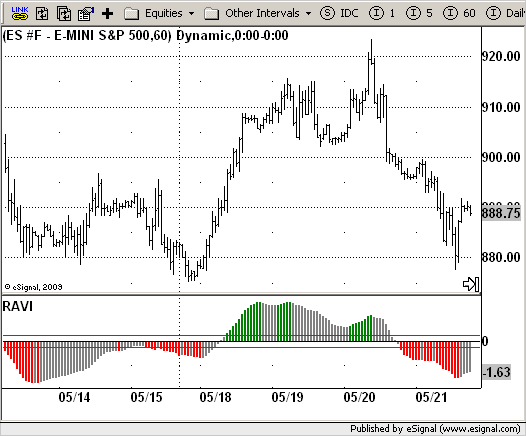# RAVI (Modification)

ICE Data Services -

RAVI.efs
EFSLibrary - Discussion Board

File Name: RAVI.efs

Description:
RAVI (Modification)For

mula Parameters:

• SMA Length 1 : 7
• SMA Length 2 : 65
• Upper Level : 0.3
• Lower Level : -0.3

Notes:
The indicator is alomost identical to the Price Oscillator and the MACD. Its uniqueness is in the usage of the exponent of the convergence-divergence of the rate as a trend indicator, which draws attention to the divergence and not to the intersection of the moving averages.
T. Chande recommends the following levels for the indicator:

• plus/minus 0.3% or plus/minus 0.1% (depending on the market).

When the indicator crosses above the upper level it is assumed that a rising trend has started.
When the indicator crosses below the lower level it is assumed that a descending trend has
started.
The rising trend continues as the RAVI line continues to rise and conversely the descending
trend continues as the RAVI continues to decrease.
Once the indicator begins to turn back to the zero line it is assumed that the trend has ended and a channel has started. If however the indicator inverts again without passing through the range between the levels it is assumed that the trend has renewed.

RAVI.efsEFS Code:

```/*********************************
Provided By:
eSignal (Copyright c eSignal), a division of Interactive Data
Formula Script (EFS) is for educational purposes only and may be
modified and saved under a new file name.  eSignal is not responsible
for the functionality once modified.  eSignal reserves the right
to modify and overwrite this EFS file with each new release.

Description:
RAVI (Modification)

Version:            1.0  06/10/2009

Formula Parameters:                     Default:
SMA Length 1                        7
SMA Length 2                        65
Upper Level                         0.3
Lower Level                         -0.3
Notes:
The indicator is alomost identical to the Price Oscillator and the MACD. Its uniqueness is
in the usage of the exponent of the convergence-divergence of the rate as a trend indicator,
which draws attention to the divergence and not to the intersection of the moving averages.
T. Chande recommends the following levels for the indicator:
plus/minus 0.3% or plus/minus 0.1% (depending on the market). When the indicator crosses
above the upper level it is assumed that a rising trend has started.
When the indicator crosses below the lower level it is assumed that a descending trend has
started.
The rising trend continues as the RAVI line continues to rise and conversely the descending
trend continues as the RAVI continues to decrease.
Once the indicator begins to turn back to the zero line it is assumed that the trend has
ended and a channel has started. If however the indicator inverts again without passing
through the range between the levels it is assumed that the trend has renewed.
**********************************/

var fpArray = new Array();
var bInit = false;

function preMain(){
setPriceStudy(false);
setShowCursorLabel(true);
setShowTitleParameters(false);
setStudyTitle("RAVI");
setCursorLabelName("RAVI", 0);
setPlotType(PLOTTYPE_HISTOGRAM, 0);
setDefaultBarFgColor(Color.grey, 0);
setDefaultBarThickness(2, 0);
var x = 0;
fpArray[x] = new FunctionParameter("SMA1_Len", FunctionParameter.NUMBER);
with(fpArray[x++]) {
setName("SMA Length 1");
setLowerLimit(1);
setDefault(7);
}
fpArray[x] = new FunctionParameter("SMA2_Len", FunctionParameter.NUMBER);
with(fpArray[x++]) {
setName("SMA Length 2");
setLowerLimit(1);
setDefault(65);
}
fpArray[x] = new FunctionParameter("LevelUp", FunctionParameter.NUMBER);
with(fpArray[x++]) {
setName("Upper Level");
setDefault(0.3);
}
fpArray[x] = new FunctionParameter("LevelDn", FunctionParameter.NUMBER);
with(fpArray[x++]) {
setName("Lower Level");
setDefault(-0.3);
}
}

var xRAVI = null;

function main(SMA1_Len, SMA2_Len, LevelUp, LevelDn) {
var nBarState = getBarState();
var nCurrent = 0;
var nPrev = 0;
if (nBarState == BARSTATE_ALLBARS) {
if(SMA1_Len == null) SMA1_Len = 7;
if(SMA2_Len == null) SMA2_Len = 65;
if(LevelUp == null) LevelUp = 0.3;
if(LevelDn == null) LevelDn = -0.3;
}
if (bInit == false) {
xRAVI = efsInternal("Calc_RAVI", SMA1_Len, SMA2_Len);
bInit = true;
}
nCurrent = xRAVI.getValue(0);
nPrev = xRAVI.getValue(-1);
if (nPrev == null) return;
if ((nCurrent > nPrev) && (nCurrent > LevelUp)) {
setBarFgColor(Color.green, 0);
}
if ((nCurrent < nPrev) && (nCurrent < LevelDn)) {
setBarFgColor(Color.red, 0);
}
return nCurrent;
}

var bSecondInit = false;
var xSMA1 = null;
var xSMA2 = null;

function Calc_RAVI(SMA1_Len, SMA2_Len) {
var nRes = 0;
var nSMA2 = 0;
var nSMA1 = 0;
if (bSecondInit == false) {
xSMA1 = sma(SMA1_Len);
xSMA2 = sma(SMA2_Len);
bSecondInit = true;
}
nSMA2 = xSMA2.getValue(0);
nSMA1 = xSMA1.getValue(0);
if (nSMA2 == null || nSMA1 == null) return;
nRes = ((nSMA1 - nSMA2) * 100) / nSMA2;
return nRes;
}```